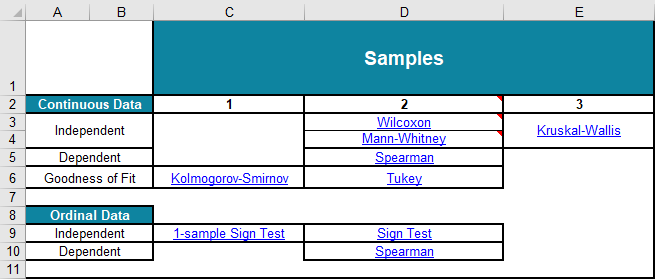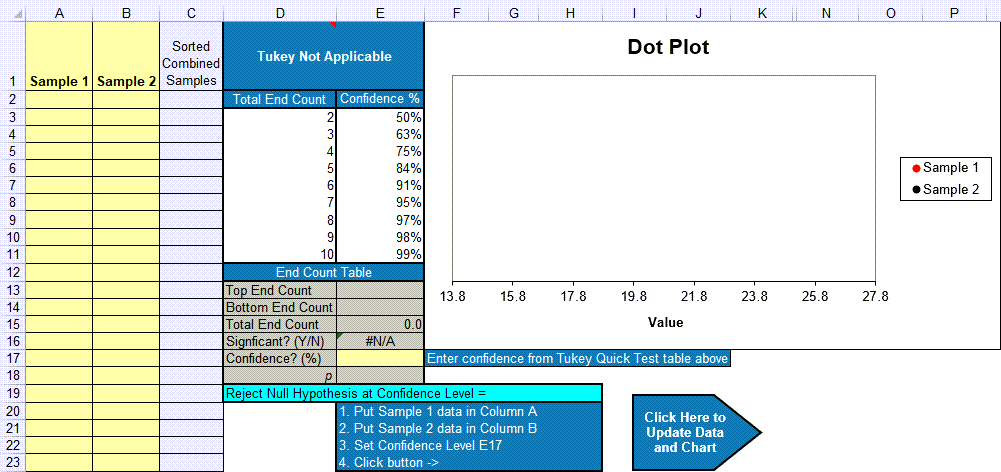# Tukey Quick Test Calculator in Excel

## Tukey Tests are Easy Using QI Macros Statistical Add-in for Excel

### QI Macros Tukey Test Template in Excel\

Note: Excel does not provide Tukey Tests; QI Macros adds this functionality.

### When to Use a Tukey Quick Test

A Tukey Quick Test is like a t-test, but it can handle nonparametric (i.e., non-normal) data. It helps determine if the means are the same or different from each other. The null hypothesis H0 is that the means are the same.

Tukey's Quick Test can be used when:

• There are two unpaired samples of similar size that overlap each other. Ratio of sizes should not exceed 4:3.
• One sample contains the highest value, the other sample contains the lowest value. One sample cannot contain both the highest and the lowest value, nor can both samples have the same high or low value.

By adding the counts of the number of unmatched points on either end, one can determine the 5%, 1% and 0.1% critical values as roughly 7, 10, and 13 points.

### Tukey Quick Test Example Using QI Macros

This example uses the data from Tukey's original paper (A Quick, Compact, Two-Sample Test To Duckworth's Specifications, Technometrics, Vol. 1, No. 1, February, 1959):

#### To run a Tukey Test in QI Macros follow these steps:

1. Click on the QI Macros menu > Stat Templates > Tukey:
2.3. A workbook will open to a worksheet with links to various Non-Parametric tests including the Tukey Test. Click on the Tukey hyper-link to go to the Tukey worksheet:4. The Tukey Quick Test template will open with some pre-populated data in columns A and B. Just delete this data and enter your own. Note, that without data, D1:E1 show "Tukey Not Applicable." If the data violates any of the rules, the template will not calculate the Tukey Quick test:
5.6. Input your data in columns A and B and confidence level in cell E17 (default = 95).
7. Next, click on the arrow in the lower right corner to Update column C and the Chart:### How End Counts (cells E13:15) are Determined

If we combine and sort the points from columns A and B, we can see that there are 5 unmatched points on either end (for a total of 10). And Tukey counts a tie (14.6 and 15.0) as 0.5. There are two of these for another count of 1. 10 + 1 equals a total of 11. This means that the two-sided confidence of 99% (>=10) applies:### Interpreting the Tukey Quick Test results

 If Then Total End Count >= 7 Reject the null hypothesis at 5% confidence level. Total End Count >= 10 Reject the null hypothesis at 1% confidence level. Total End Count >= 13 Reject the null hypothesis at 0.1% confidence level. Total End Count < 7 Cannot Reject the null hypothesis (accept the null hypothesis)

The null hypothesis H0 is that the means are the same. In this example, since the End Count = 11, we reject the null hypothesis at a 1% confidence level.

### Why Choose QI Macros Statistical Software for Excel?### Affordable

• Only \$329 USD - less with quantity discounts
• No annual fees
• Free Technical Support### Easy to Use

• Works Right in Excel
• Interprets Results for You
• Accurate Results Without Worry### Proven and Trusted

• 100,000 Users in 80 Countries
• Celebrating 20th Anniversary
• Five Star CNET Rating - Virus Free